# 标签： 深度学习

## 概况

• 天元 MegEngine 官方网站：https://megengine.org.cn/
• GitHub repo：https://github.com/MegEngine/MegEngine
• 中文社区：https://discuss.megengine.org.cn/
• MegStudio：https://studio.brainpp.com/

## 安装

• 64 位、16.04 及以上版本的 Ubuntu
• Python 3.5+
• NVIDIA 驱动版本 418.x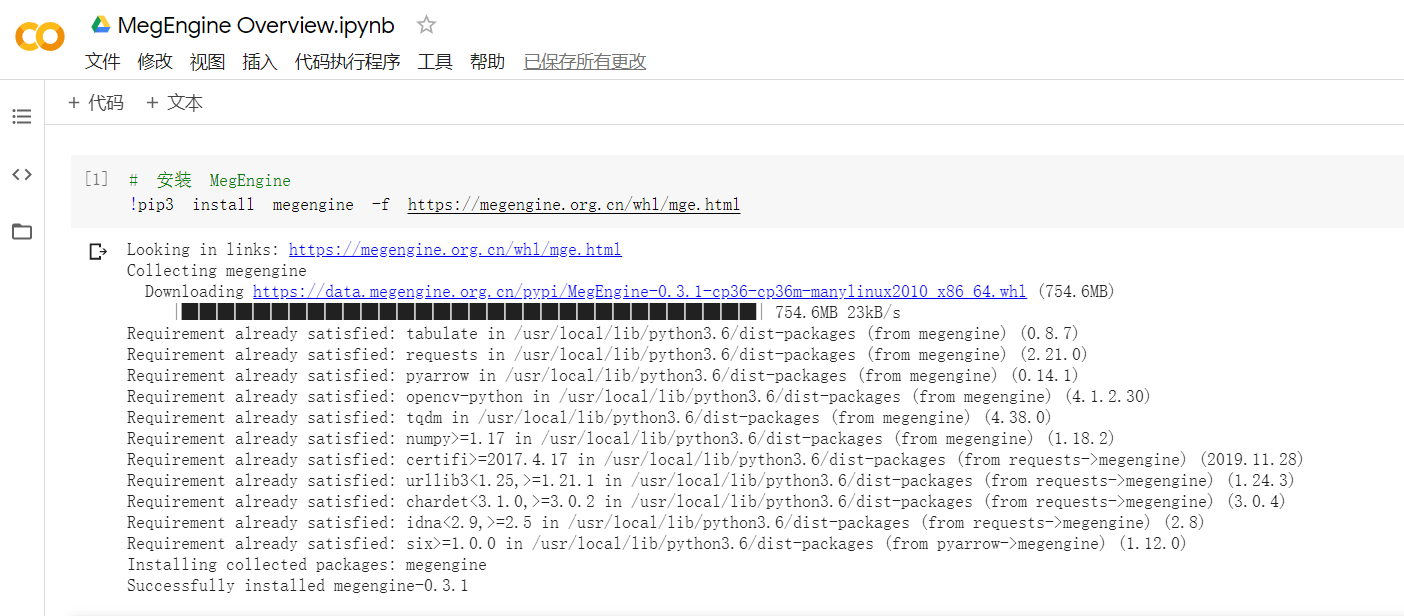MegEngine 安装包本身集成了 CUDA 环境，因此不区分 CPU 和 GPU 版本。

pip3 install megengine -f https://megengine.org.cn/whl/mge.html

import 一下来测试安装是否成功，官方对 MegEngine 的习惯性缩写是 mge

import megengine as mge

## 体验

### MegStudio

MegStudio 是旷视开放的提供免费算力的在线深度学习开发平台。目前提供下面三种配置的环境，其中基础版的环境不限时长，高级版（CPU）和高级版（GPU）环境需要通过算力卡获得。目前算力卡是通过邀请用户的方式获得。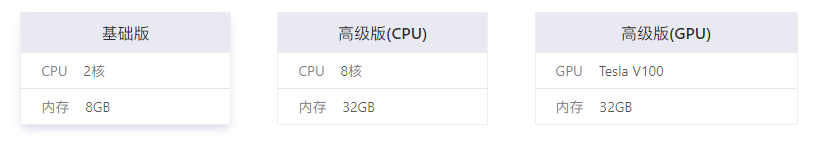MegStudio 开发环境是基于 JupyterLab，环境关闭之后额外添加的文件会被销毁。使用体验上和 JupyterLab 基本没有区别，如果官方能默认支持一下代码补全就更好了。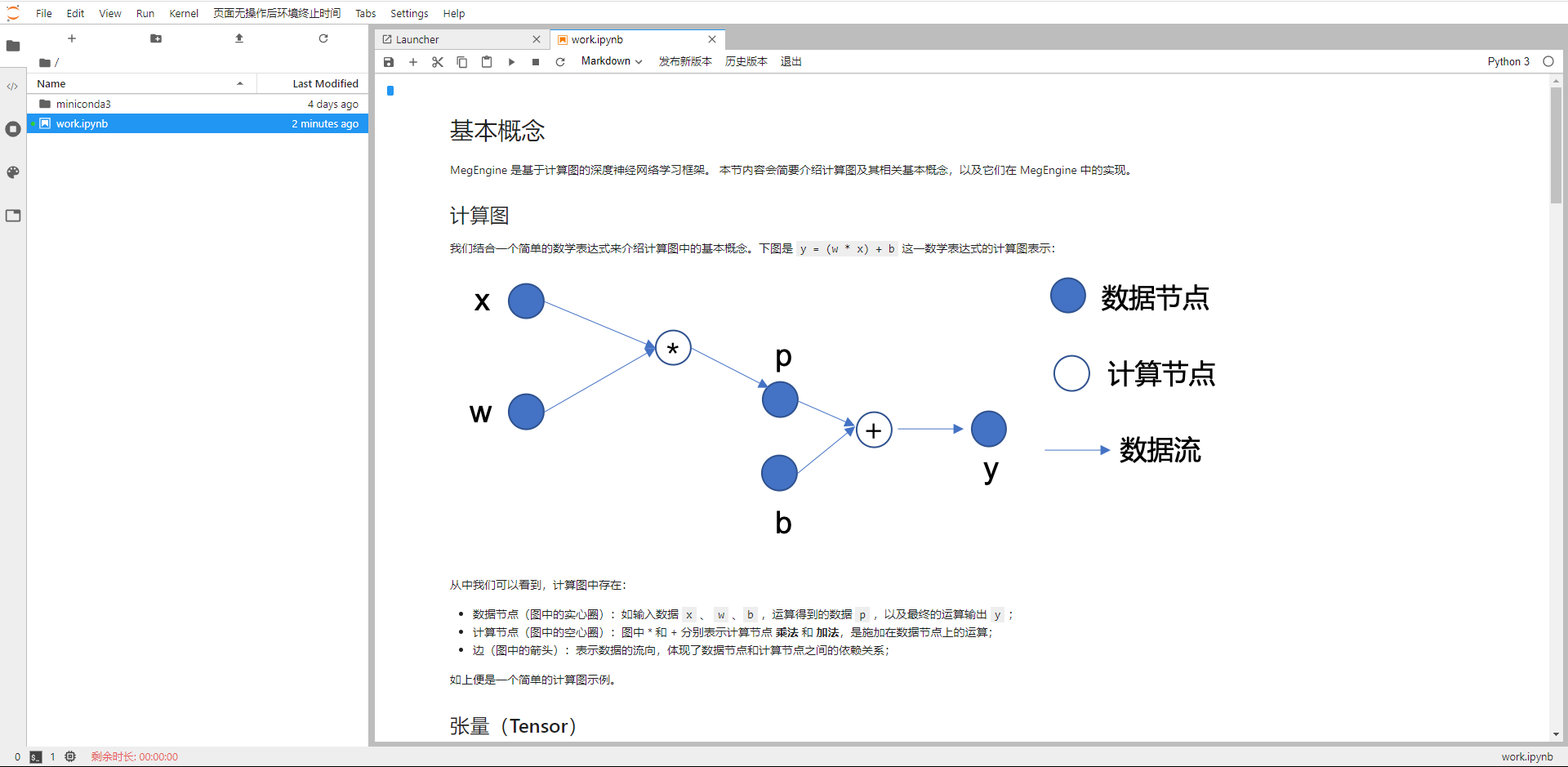### 友好的中文文档MegEngine 的文档中穿插了对神经网络知识的简要讲解，比如下面是文档中介绍的 BP 过程。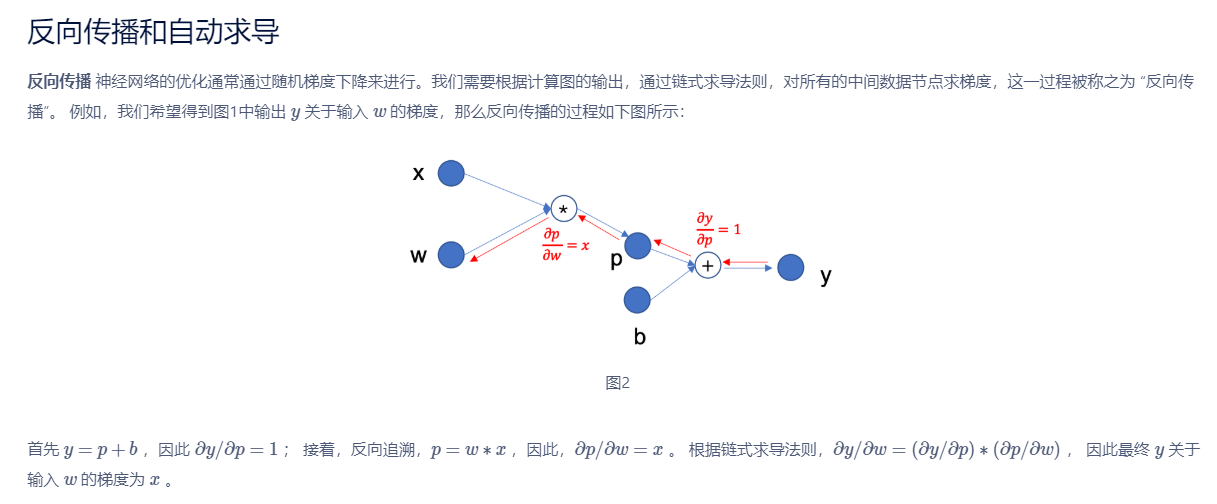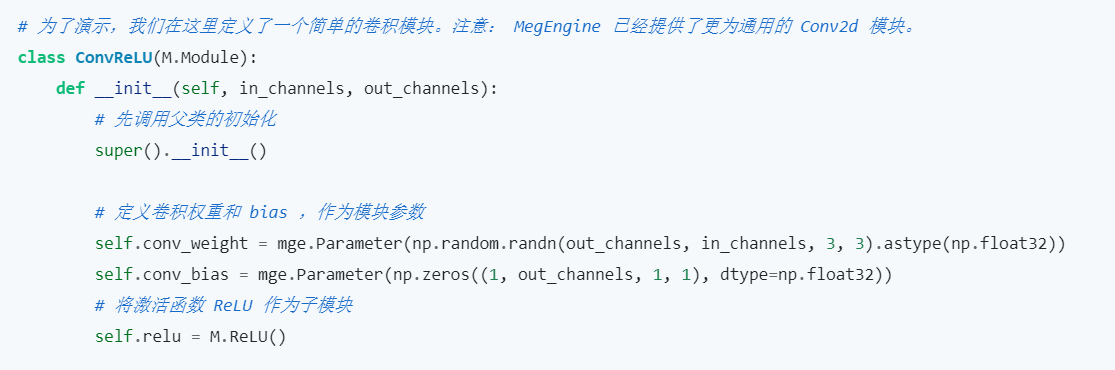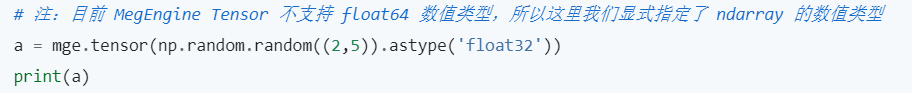### 支持基于 Module 的网络搭建

MegEngine 提供两种网络搭建方式：基于 functional （提供常见算子）和基于 Module（提供常见网络层）。

# MegEngine Implementation
class LeNet(M.Module):
def __init__(self):
super(LeNet, self).__init__()
self.conv1 = M.Conv2d(1, 6, 5)
self.relu1 = M.ReLU()
self.pool1 = M.MaxPool2d(2, 2)
self.conv2 = M.Conv2d(6, 16, 5)
self.relu2 = M.ReLU()
self.pool2 = M.MaxPool2d(2, 2)
self.fc1 = M.Linear(16 * 5 * 5, 120)
self.relu3 = M.ReLU()
self.fc2 = M.Linear(120, 84)
self.relu4 = M.ReLU()
self.classifer = M.Linear(84, 10)

def forward(self, x):
x = self.pool1(self.relu1(self.conv1(x)))
x = self.pool2(self.relu2(self.conv2(x)))
x = F.flatten(x, 1)
x = self.relu3(self.fc1(x))
x = self.relu4(self.fc2(x))
x = self.classifer(x)
return x

# PyTorch Implementation
class LeNet5(nn.Module):
def __init__(self):
super().__init__()
self.conv1 = nn.Conv2d(1, 6, 5, padding=2)
self.conv2 = nn.Conv2d(6, 16, 5)
self.fc1 = nn.Linear(16*5*5, 120)
self.fc2 = nn.Linear(120, 84)
self.fc3 = nn.Linear(84, 10)
def forward(self, x):
x = F.max_pool2d(F.relu(self.conv1(x)), (2, 2))
x = F.max_pool2d(F.relu(self.conv2(x)), (2, 2))
x = x.view(-1, self.num_flat_features(x))
x = F.relu(self.fc1(x))
x = F.relu(self.fc2(x))
x = self.fc3(x)
return x
def num_flat_features(self, x):
size = x.size()[1:]
num_features = 1
for s in size:
num_features *= s
return num_features


### 动态图与静态图的转换

import megengine.functional as F
from megengine.jit import trace

trace.enabled = True

@trace
def train_func(data, label, *, opt, net):
pred = net(data)
loss = F.cross_entropy_with_softmax(pred, label)
opt.backward(loss)
return pred, loss

train_func(data, label, opt=optimizer, net=le_net)



### 中文社区MegEngine 建立了中文语言的 社区，对国内开发者无疑是一大好处，我在站务反馈区提了一个捉虫帖，没想到没几分钟之后管理员就反馈修改完毕，效率可以说是非常高了！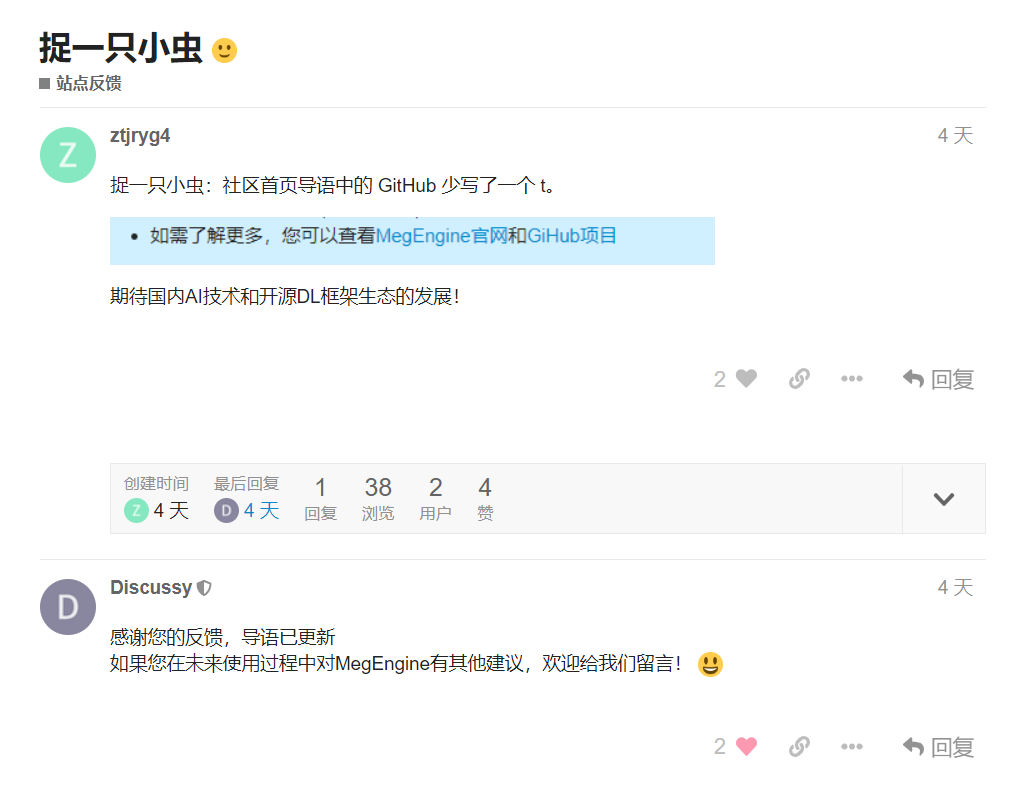1. PyTorch概览
3. 神经网络
4. 训练一个分类器

# 数据

• 对于图像，Pillow，OpenCV等软件包很有用
• 对于音频，可以使用scipy和librosa等包
• 对于文本，基于Python或Cython的加载、NLTK和SpaCy都很有用# 训练图像分类器

1. 使用以下命令加载和标准化CIFAR10训练和测试数据集 torchvision
2. 定义卷积神经网络
3. 定义损失函数
4. 根据训练数据训练网络
5. 在测试数据上测试网络

## 1. 加载CIFAR10并将其规则化

torchvision的帮助下，加载CIFAR10非常容易。

import torch
import torchvision
import torchvision.transforms as transforms


torchvision数据集的输出是在[0,1]范围的PILImage。我们将它们转换成范围是[-1,1]的张量。

Note:

transform = transforms.Compose(
[transforms.ToTensor(),
transforms.Normalize((0.5, 0.5, 0.5), (0.5, 0.5, 0.5))])

trainset = torchvision.datasets.CIFAR10(root='./data', train=True,
shuffle=True, num_workers=2)

testset = torchvision.datasets.CIFAR10(root='./data', train=False,
shuffle=False, num_workers=2)

classes = ('plane', 'car', 'bird', 'cat',
'deer', 'dog', 'frog', 'horse', 'ship', 'truck')

Files already downloaded and verified


import matplotlib.pyplot as plt
import numpy as np

# functions to show an image

def imshow(img):
img = img / 2 + 0.5     # unnormalize
npimg = img.numpy()
plt.imshow(np.transpose(npimg, (1, 2, 0)))
plt.show()

# get some random training images
images, labels = dataiter.next()

# show images
imshow(torchvision.utils.make_grid(images))
# print labels
print(' '.join('%5s' % classes[labels[j]] for j in range(4)))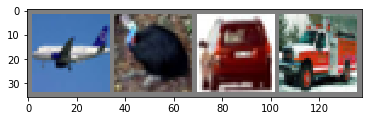plane  bird   car truck


## 2. 定义卷积神经网络

import torch.nn as nn
import torch.nn.functional as F

class Net(nn.Module):
def __init__(self):
super(Net, self).__init__()
self.conv1 = nn.Conv2d(3, 6, 5)
self.pool = nn.MaxPool2d(2, 2)
self.conv2 = nn.Conv2d(6, 16, 5)
self.fc1 = nn.Linear(16 * 5 * 5, 120)
self.fc2 = nn.Linear(120, 84)
self.fc3 = nn.Linear(84, 10)

def forward(self, x):
x = self.pool(F.relu(self.conv1(x)))
x = self.pool(F.relu(self.conv2(x)))
x = x.view(-1, 16 * 5 * 5)
x = F.relu(self.fc1(x))
x = F.relu(self.fc2(x))
x = self.fc3(x)
return x

net = Net()


## 3. 定义损失函数和优化器

import torch.optim as optim

criterion = nn.CrossEntropyLoss()
optimizer = optim.SGD(net.parameters(), lr=0.001, momentum=0.9)


## 4. 训练网络

for epoch in range(2):  # loop over the dataset multiple times

running_loss = 0.0
for i, data in enumerate(trainloader, 0):
# get the inputs; data is a list of [inputs, labels]
inputs, labels = data

# forward + backward + optimize
outputs = net(inputs)
loss = criterion(outputs, labels)
loss.backward()
optimizer.step()

# print statistics
running_loss += loss.item()
if i % 2000 == 1999:    # print every 2000 mini-batches
print('[%d, %5d] loss: %.3f' %
(epoch + 1, i + 1, running_loss / 2000))
running_loss = 0.0

print('Finished Training')

[1,  2000] loss: 2.192
[1,  4000] loss: 1.815
[1,  6000] loss: 1.630
[1,  8000] loss: 1.541
[1, 10000] loss: 1.475
[1, 12000] loss: 1.446
[2,  2000] loss: 1.370
[2,  4000] loss: 1.350
[2,  6000] loss: 1.302
[2,  8000] loss: 1.292
[2, 10000] loss: 1.268
[2, 12000] loss: 1.253
Finished Training


## 5. 在测试集上测试网络

dataiter = iter(testloader)
images, labels = dataiter.next()

# print images
imshow(torchvision.utils.make_grid(images))
print('GroundTruth: ', ' '.join('%5s' % classes[labels[j]] for j in range(4)))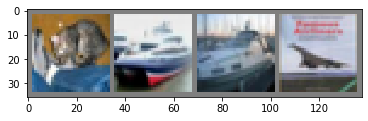GroundTruth:    cat  ship  ship plane


outputs = net(images)


outputs给出十个类的置信度，置信度越高，代表网络认为这张图片越可能属于这一类别，所以我们现在获取置信度最高的类别的下标。

_, predicted = torch.max(outputs, 1)

print('Predicted: ', ' '.join('%5s' % classes[predicted[j]]
for j in range(4)))

Predicted:    cat  ship  ship  ship


correct = 0
total = 0
images, labels = data
outputs = net(images)
_, predicted = torch.max(outputs.data, 1)
total += labels.size(0)
correct += (predicted == labels).sum().item()

print('Accuracy of the network on the 10000 test images: %d %%' % (
100 * correct / total))

Accuracy of the network on the 10000 test images: 53 %


class_correct = list(0. for i in range(10))
class_total = list(0. for i in range(10))
images, labels = data
outputs = net(images)
_, predicted = torch.max(outputs, 1)
c = (predicted == labels).squeeze()
for i in range(4):
label = labels[i]
class_correct[label] += c[i].item()
class_total[label] += 1

for i in range(10):
print('Accuracy of %5s : %2d %%' % (
classes[i], 100 * class_correct[i] / class_total[i]))

Accuracy of plane : 57 %
Accuracy of   car : 58 %
Accuracy of  bird : 57 %
Accuracy of   cat : 49 %
Accuracy of  deer : 49 %
Accuracy of   dog : 19 %
Accuracy of  frog : 41 %
Accuracy of horse : 67 %
Accuracy of  ship : 73 %
Accuracy of truck : 61 %


# 在GPU上训练

device = torch.device("cuda:0" if torch.cuda.is_available() else "cpu")

# Assuming that we are on a CUDA machine, this should print a CUDA device:

print(device)

cuda:0


net.to(device)

Net(
(conv1): Conv2d(3, 6, kernel_size=(5, 5), stride=(1, 1))
(pool): MaxPool2d(kernel_size=2, stride=2, padding=0, dilation=1, ceil_mode=False)
(conv2): Conv2d(6, 16, kernel_size=(5, 5), stride=(1, 1))
(fc1): Linear(in_features=400, out_features=120, bias=True)
(fc2): Linear(in_features=120, out_features=84, bias=True)
(fc3): Linear(in_features=84, out_features=10, bias=True)
)


inputs, labels = data.to(device), data.to(device)


* 全面了解PyTorch的Tensor库和神经网络。
* 训练一个小型神经网络对图像进行分类。

1. PyTorch概览
3. 神经网络
4. 训练一个分类器# 定义网络

import torch
import torch.nn as nn
import torch.nn.functional as F

class Net(nn.Module):

def __init__(self):
super(Net, self).__init__() # 多重继承
# 一个图像输入channel，六个输出channel，3*3卷积核
self.conv1 = nn.Conv2d(1,6,3)
self.conv2 = nn.Conv2d(6,16,3)
# 线性变换: y = Wx + b
self.fc1 = nn.Linear(16 * 6 * 6, 120)  # 6*6 from image dimension
self.fc2 = nn.Linear(120, 84)
self.fc3 = nn.Linear(84, 10)

def forward(self,x):
# 经过卷积层1、relu激活函数、max_pool降采样
x = F.max_pool2d(F.relu(self.conv1(x)),(2,2))
# 经过卷积层2、relu激活函数、max_pool降采样
# 如果降采样尺寸为正方形，也可以只写一个数字
x = F.max_pool2d(F.relu(self.conv2(x)), 2)
# resize
x = x.view(-1, self.num_flat_features(x))
# 线性变换
x = F.relu(self.fc1(x))
x = F.relu(self.fc2(x))
x = self.fc3(x)
return x

def num_flat_features(self, x):
size = x.size()[1:]  # all dimensions except the batch dimension
num_features = 1
for s in size:
num_features *= s
return num_features

net = Net()
print(net)

Net(
(conv1): Conv2d(1, 6, kernel_size=(3, 3), stride=(1, 1))
(conv2): Conv2d(6, 16, kernel_size=(3, 3), stride=(1, 1))
(fc1): Linear(in_features=576, out_features=120, bias=True)
(fc2): Linear(in_features=120, out_features=84, bias=True)
(fc3): Linear(in_features=84, out_features=10, bias=True)
)


params = list(net.parameters())
print(len(params))
print(params.size())  # conv1's .weight

10
torch.Size([6, 1, 3, 3])


input = torch.randn(1, 1, 32, 32)
out = net(input)
print(out)

tensor([[ 0.0203,  0.0255,  0.0466,  0.1283,  0.1069,  0.1514, -0.0276, -0.0390,


net.zero_grad()
out.backward(torch.randn(1, 10))


Note:

torch.nn仅支持mini-batches。整个torch.nn仅支持作为mini-batch采样的输入，而非单采样的输入。

• torch.Tensor – 一个支持例如backward()等autograd操作的多维数组，同时包含关于张量的梯度。
• nn.Module – 神经网络模块。一个封装参数的便捷途径，同时有将其移动到GPU、导出、加载的帮助器。
• nn.Parameter – 一种张量，当给Module赋值时能够自动注册为一个参数。
• autograd.Function – 使用autograd自动实现前向传播和反向传播。每个张量的操作都至少会生成一个独立的Function节点，与生成该张量的函数相连之后，记录下操作历史。

* 如何定义一个神经网络
* 处理输入并调用反向传播

* 计算损失函数
* 更新网络权重

# 损失函数

nn包中有几个不同的损失函数。一个简单的损失函数是：nn.MSELoss，计算输入和目标之间的均方误差。

output = net(input)
target = torch.randn(10)  # a dummy target, for example
target = target.view(1, -1)  # make it the same shape as output
criterion = nn.MSELoss()

loss = criterion(output, target)
print(loss)

tensor(0.5661, grad_fn=<MseLossBackward>)


::

input -> conv2d -> relu -> maxpool2d -> conv2d -> relu -> maxpool2d
-> view -> linear -> relu -> linear -> relu -> linear
-> MSELoss
-> loss


print(loss.grad_fn)  # MSELoss

<MseLossBackward object at 0x0000014700F28940>


# 反向传播

net.zero_grad()     # zeroes the gradient buffers of all parameters

loss.backward()


conv1.bias.grad before backward
tensor([0., 0., 0., 0., 0., 0.])
tensor([-0.0008, -0.0017, -0.0009,  0.0055, -0.0086,  0.0021])


• 如何更新网络权重

# 更新网络权重

weight = weight - learning_rate * gradient

learning_rate = 0.01
for f in net.parameters():


import torch.optim as optim

# 创建你的优化器
optimizer = optim.SGD(net.parameters(), lr=0.01)

# 在训练循环中
output = net(input)
loss = criterion(output, target)
loss.backward()
optimizer.step()    # Does the update


1. PyTorch概览
3. 神经网络
4. 训练一个分类器

autograd模块为张量的所有操作提供自动微分。这是一个由运行定义的框架，这意味着你的反向传播是由代码的运行的方式定义的，并且每一次迭代都可能不同。

# Tensor

torch.Tensor是这个包的核心类。如果将.requires_grad属性设置为True，这将开始追踪它上的所有操作。当计算结束，你可以调用.backward()方法，自动计算所有梯度。该张量的梯度将累加到.grad属性中。

TensorFunction相互连接并建立一个无环图，该图对完整的计算历史进行编码。每个张量都有一个.grad_fn属性，该属性引用一个创建这个张量的Function（用户创建的张量除外，他们的.grad_fn属性为None）。

import torch


x = torch.ones(2, 2, requires_grad=True)
print(x)

tensor([[1., 1.],


y = x + 2
print(y)

tensor([[3., 3.],


y是由相加操作得来的张量，所以它有grad_fn属性。

print(y.grad_fn)

<AddBackward0 object at 0x0000022497B4C630>


z = y * y * 3
out = z.mean()

print(z, out)

tensor([[27., 27.],


.requires_grad_( ... )方法可以变换一个张量的requires_grad属性。

a = torch.randn(2, 2)
a = ((a * 3) / (a - 1))
b = (a * a).sum()

b = (a * a).sum()

False
None
True
<SumBackward0 object at 0x0000022497F12EF0>


out.backward()


print(x.grad)

tensor([[4.5000, 4.5000],
[4.5000, 4.5000]])


x = torch.randn(3, requires_grad=True)

y = x * 2
print(y)
while y.data.norm() < 1000:
y = y * 2
print(y)

tensor([-0.1796, -0.6436, -1.6582], grad_fn=<MulBackward0>)


v = torch.tensor([0.1, 1.0, 0.0001], dtype=torch.float)
y.backward(v)


tensor([2.0480e+02, 2.0480e+03, 2.0480e-01])


print(x.requires_grad)


True
True
False


1. PyTorch概览
3. 神经网络
4. 训练一个分类器

# Tensor 张量

Tensor类似于Numpy的ndarray，但不同的是，Tensor可以在GPU上被加速。

from __future__ import print_function
import torch


x = torch.empty(5,3)
print(x)

tensor([[0., 0., 0.],
[0., 0., 0.],
[0., 0., 0.],
[0., 0., 0.],
[0., 0., 0.]])


x = torch.rand(5,3)
print(x)

tensor([[0.4907, 0.1470, 0.9752],
[0.9518, 0.6973, 0.4775],
[0.5643, 0.6586, 0.3142],
[0.2364, 0.8435, 0.6187],
[0.3253, 0.5903, 0.9939]])


x = torch.zeros(5,3,dtype=torch.long)
print(x)

tensor([[0, 0, 0],
[0, 0, 0],
[0, 0, 0],
[0, 0, 0],
[0, 0, 0]])


x = torch.tensor([6.7,6,7])
print(x)

tensor([6.7000, 6.0000, 7.0000])


print('x:\n',x)
y = x.new_ones(5, 3, dtype=torch.double)
print('y:\n',y)

x = torch.randn_like(x, dtype=torch.float)    # dtype被改写，但是结果继承了size。
print(x)

x:
tensor([6.7000, 6.0000, 7.0000])
y:
tensor([[1., 1., 1.],
[1., 1., 1.],
[1., 1., 1.],
[1., 1., 1.],
[1., 1., 1.]], dtype=torch.float64)
tensor([-0.3186,  0.5506,  0.4940])


print(x.size())

torch.Size()


# Operations 操作

## 相加

PyTorch中有许多种相加语法：

x = torch.rand(5, 3)
y = torch.rand(5, 3)
print(x + y)

tensor([[0.9943, 0.3976, 0.6645],
[0.6796, 1.1703, 1.2389],
[1.5879, 1.1208, 1.5912],
[1.2165, 0.8195, 1.0364],
[1.2178, 1.3195, 1.1736]])


print(torch.add(x,y))

tensor([[0.9943, 0.3976, 0.6645],
[0.6796, 1.1703, 1.2389],
[1.5879, 1.1208, 1.5912],
[1.2165, 0.8195, 1.0364],
[1.2178, 1.3195, 1.1736]])


result = torch.empty(5,3)
print(result)

tensor([[0.9943, 0.3976, 0.6645],
[0.6796, 1.1703, 1.2389],
[1.5879, 1.1208, 1.5912],
[1.2165, 0.8195, 1.0364],
[1.2178, 1.3195, 1.1736]])


y.add_(x)
print(y)

tensor([[0.9943, 0.3976, 0.6645],
[0.6796, 1.1703, 1.2389],
[1.5879, 1.1208, 1.5912],
[1.2165, 0.8195, 1.0364],
[1.2178, 1.3195, 1.1736]])


Note:

## 索引

NumPy中的索引方法可以使用：

print(x[:, 1])

tensor([0.0200, 0.4981, 0.2466, 0.7497, 0.8315])


## Resizing

x = torch.randn(4, 4)
y = x.view(16)
z = x.view(-1, 8)  # the size -1 is inferred from other dimensions
print(x.size(), y.size(), z.size())

torch.Size([4, 4]) torch.Size() torch.Size([2, 8])


## 取值

x = torch.randn(1)
print(x)
print(x.item())

tensor([-0.7093])
-0.709314227104187


# 与NumPy的沟通桥梁

Torch张量将会和NumPy数组共享同一个内存位置（Torch张量在CPU上的情况下），其中一个发生改变，另一个也会随之改变。

## 将Torch Tensor转换为NumPy数组

a = torch.ones(5)
print(a)
b = a.numpy()
print(b)
print(a)
print(b)

tensor([1., 1., 1., 1., 1.])
[1. 1. 1. 1. 1.]
tensor([2., 2., 2., 2., 2.])
[2. 2. 2. 2. 2.]


## 将NumPy数组转换为Torch Tensor

import numpy as np
a = np.ones(5)
b = torch.from_numpy(a)
print(a)
print(b)

[2. 2. 2. 2. 2.]
tensor([2., 2., 2., 2., 2.], dtype=torch.float64)


# CUDA Tensors

if torch.cuda.is_available():
device = torch.device("cuda")          # 创建CUDA设备对象
y = torch.ones_like(x, device=device)  # 直接在GPU上
x = x.to(device)
# x = x.to("cuda") 也可以这么写
z = x + y
print(z)
print(z.to("cpu", torch.double))       # .to 方法可以同时转换数据类型

tensor([0.2907], device='cuda:0')
tensor([0.2907], dtype=torch.float64)